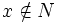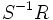# Nilradical equals intersection of all prime ideals

## Statement

In a commutative unital ring, the nilradical (defined as the smallest radical ideal, or as the set of nilpotent elements) is the intersection of all prime ideals.

## Proof

### Hands-on proof

We need to show that if$R$ is a commutative unital ring with nilradical$N$, and if$x \notin N$, then there exists a prime ideal not containing$x$. Consider the multiplicatively closed subset$S$ comprising$1$ and the positive powers of$x$. Then, the following can be verified:

• There exist ideals maximal with respect to the property of intersecting$S$ trivially (this involves appealing to Zorn's lemma or an equivalent)
• Any such ideal must be a prime ideal

Thus, we have found a prime ideal not containing$x$.

### Proof using localizations

A conceptually more general version of the above is described as follows. With the same notation as before, let$S^{-1}R$ denote the localization at the multiplicative subset$S$. The ring$S^{-1}R$ has a maximal ideal (because every proper ideal is contained in a maximal ideal). The contraction of this to$R$ gives a prime ideal (because maximal ideals are prime and the contraction of a prime ideal is prime) and it is disjoint from$S$.

The two proofs are exactly the same, though the proof using localization makes things conceptually easier. In the latter proof, we hide Zorn's lemma behind the fact that every proper ideal is contained in a maximal ideal.